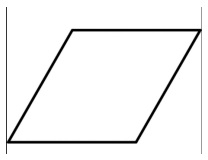# Program to find the perimeter of a rhombus using diagonals

CServer Side ProgrammingProgramming

Rhombus is a simple quadrilateral whose four sides all have the same length. And perimeter of rhombus can be found by two methods.

2. Using the diagonals

A quadrilateral has two diagonal and based on the length of diagonals the area and perimeter of the quadrilateral can be found.

To find the perimeter of a rhombus using its diagonals is 2{√(d1)2 + (d2)2 }LOGIC − To find the perimeter of a rhombus using its diagonals. You need the formula 2{√(d1)2 + (d2)2 } for this in your code you need to use the math class that supports the use of squareRoot and square of the number.

The Below code display Program to find the perimeter of rhombus using its diagonals.

## Example

#include <stdio.h>
#include <math.h>
int main(){
int d1 = 3, d2= 4, perimeter;
perimeter = (2 * sqrt(pow(d1,2)+pow(d2,2)));
printf("perimeter is %d", perimeter);
return 0;
}

## Output

perimeter is 10